Author: Oscar Cronquist Article last updated on May 05, 2022

####This article demonstrates how to use the XLOOKUP function in Excel 365.

## 1. XLOOKUP function - Overview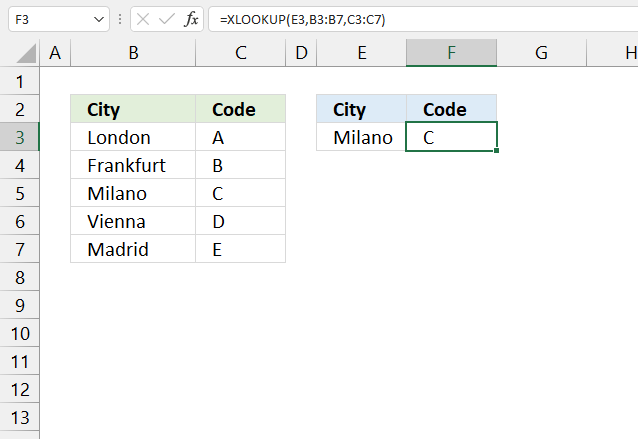The XLOOKUP function lets you search one column for a search value, and return a corresponding value in another column from the same row.

Formula in cell F2:

=XLOOKUP(E3, B3:B7, C3:C7)

The image above demonstrates the XLOOKUP function in cell F2, it uses a search value specified in cell E3 and searches column B.

A match is found in cell B5 and the corresponding value from column C is returned in cell F3.

## 2. XLOOKUP Function Syntax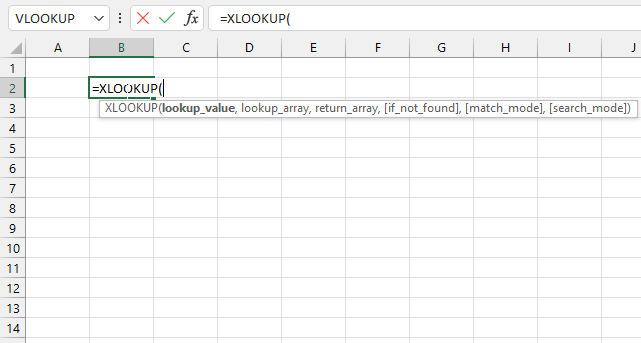XLOOKUP(lookup_value, lookup_array, return_array, [if_not_found], [match_mode], [search_mode])

## 3. XLOOKUP Function Arguments

 lookup_value Required. The value you want to look up. lookup_array Required. This is a cell range or an array that you want to search. return_array Required. This is a cell range or an array that you want to return a result from. [if_not_found] Optional. A given text string is returned if a valid match is not found. #N/A is returned if this argument is omitted and a valid match is not found. [match_mode] Optional. Match setting: 0 - Exact match. Return #N/A if no valid match is found. (Default) -1 - Exact match. Return the next smaller item if no valid match is found. 1 - Exact match. Return the next larger item if no valid match is found. 2 - Wildcard match * - Any number of characters. Example, *son matches both "Johnson" and "Wilson" but not "Jones". ? - Any single character. Example, ?n matches both "in" and "on" but not "off". ~ (tilde) followed by ?, *, or ~ - Example, How~? matches How?. [search_mode] Optional. Search setting: 1 - Search starting at the first item. (Default) -1 - Reverse search starting at the last item. 2 - Binary search, lookup_array must be sorted in ascending order. -2 - Binary search, lookup_array must be sorted in descending order.

## 5. How to return multiple values - XLOOKUP function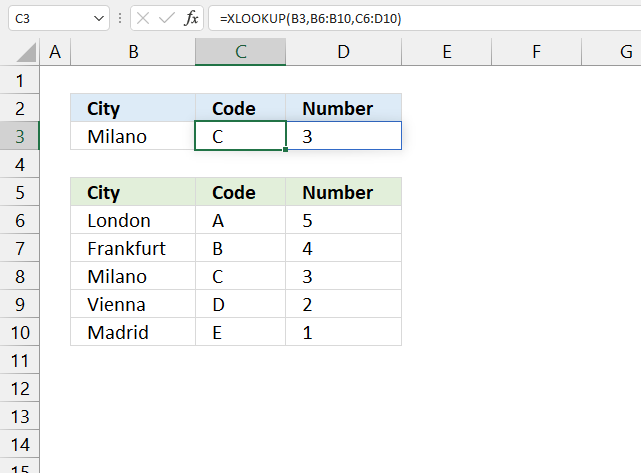The formula below demonstrates the XLOOKUP function returning more values from the same row.

Formula in cell C6:

=XLOOKUP(B6, C2:G2, C3:G3)

The return_array (3rd argument) contains two columns in this example. The lookup value is found in cell B8 and the corresponding values are in cell range C8:D8.

Note, the XLOOKUP function does not return multiple matches. It only returns the first valid match even if there are more valid matches.

I recommend that you use the FILTER function if you need to search for multiple values and return multiple corresponding values.

## 6. How to search horizontally - XLOOKUP function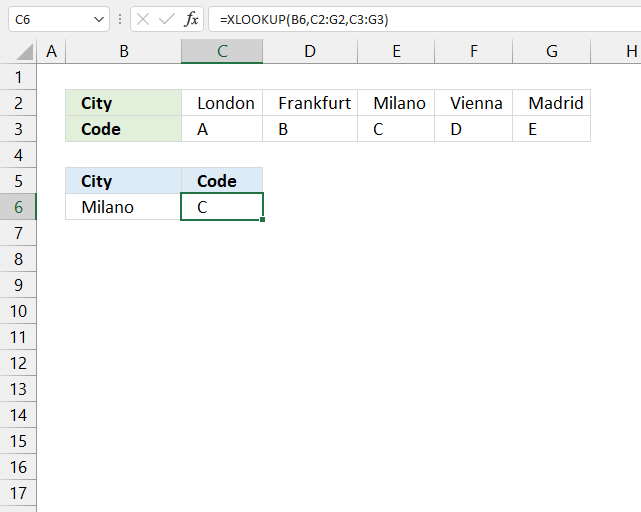This example demonstrates how to lookup a value horizontally using the XLOOKUP function and return the corresponding value from the same column.

Search value "Milano" is found in cell E2 and the formula returns value "C" from row three in the same column.

Formula in cell C6:

=XLOOKUP(B6, C2:G2, C3:G3)

## 7. How to find the last match - reverse search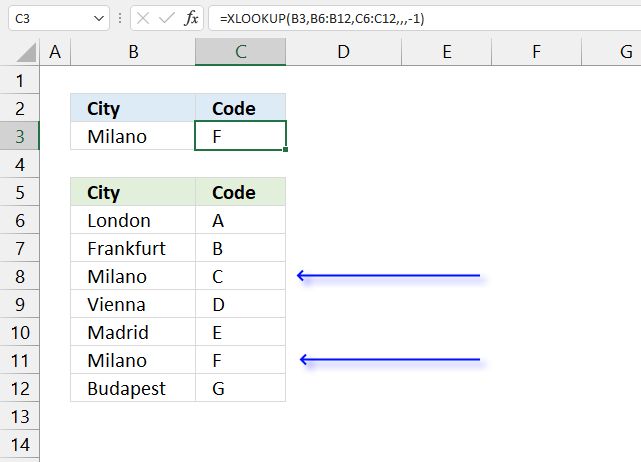The image above shows the XLOOKUP function in cell C3, it searches cell range B6:B12 starting at the last value.

There are two matches cells B8 and B11, however, the search starts at the last value and the first matching value is in cell B11. The corresponding value is in cell C11.

Formula in cell C3:

=XLOOKUP(B3,B6:B12,C6:C12,,,-1)

The last argument lets you choose the search mode.

XLOOKUP(lookup_valuelookup_arrayreturn_array, [if_not_found], [match_mode], [search_mode])

-1 : Reverse search starting at the last item.

This article explains how to do it in earlier Excel versions: Find last matching value in an unsorted table

## 8. How to do a binary xlookup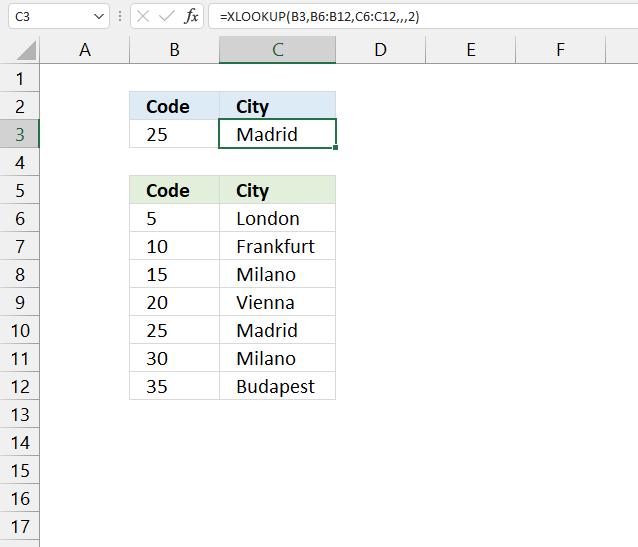This example demonstrates how to do a binary lookup using the XLOOKUP function.

Make sure the lookup_array is sorted appropriately based on the chosen value in the last argument.

Formula in cell C3:

=XLOOKUP(B3,B6:B12,C6:C12,,,-1)

The last argument lets you choose the search mode.

XLOOKUP(lookup_valuelookup_arrayreturn_array, [if_not_found], [match_mode], [search_mode])

2 : Binary search, lookup_array must be sorted in ascending order.

## 9. How to do a wildcard xlookup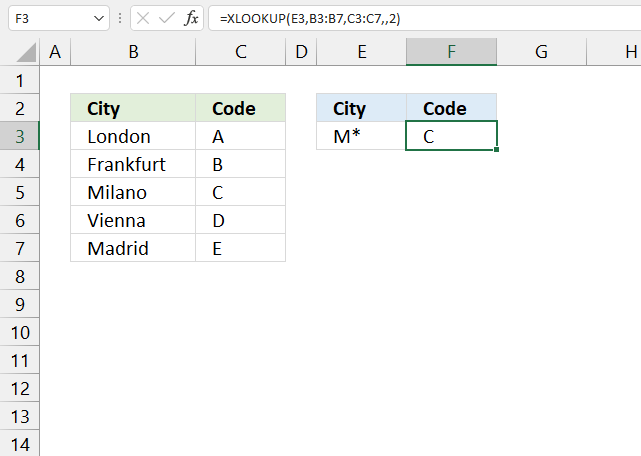The image above demonstrates how to do a wildcard lookup using the XLOOKUP function.

The asterisk matches any number of characters. M* matches both Milano and Madrid, however, Milano is the first match in the list. The corresponding value is "C" and that is returned in cell F3.

Formula in cell C3:

=XLOOKUP(B3,B6:B12,C6:C12,,2)

The second last argument lets you choose the match mode.

XLOOKUP(lookup_valuelookup_arrayreturn_array, [if_not_found], [match_mode], [search_mode])

* - Any number of characters.

? - Any single character.

~ (tilde) followed by ?, *, or ~